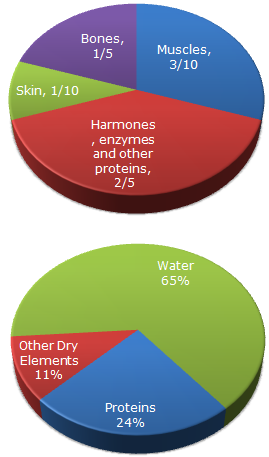# Data Interpretation - Pie Charts

### Exercise :: Pie Charts - Pie Chart 3

The following pie chart give the information about the distribution of weight in the human body according to different kinds of components. Study the pie charts and answer the question.

Distribution of Weight in Human Body1.

What percentage of proteins of the human body is equivalent to the weight of its skin ?

 A. 41.66 % B. 43.33 % C. 44.44 % D. Cannot be determined

Explanation:

Total percentage = (10 / 24) x 100 = 41.6666667 %

2.

How much of the human body is neither made of bones or skin ?

 A. 40 % B. 50 % C. 60 % D. 70 %

Explanation:

20 + 10 = 30% is made up of either bones or skin. Hence, 70% is made up of neither.

3.

What is the ratio of the distribution of proteins in the muscles to that of the distribution of proteins in the bones ?

 A. 2:1 B. 2:3 C. 3:2 D. Cannot be determined